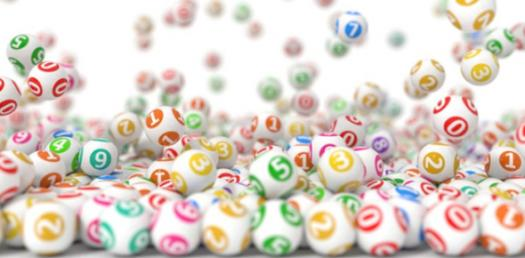The Random Quiz 1.0

5 Questions | Total Attempts: 24SettingsJust as the name suggests, the quiz below comprises of completely random questions on general knowledge to jog your memory and mind. Take it up and see how good you are with general knowledge. All the best.

• 1.
How was the whale on a stick inspired?
• A.

A cartoon whale

• B.

Cabeza de vaca

• C.

A video game

• D.

A marshmallow on a stick

• 2.
What does algebrated mean?
• A.

A wrong mathematical equation

• B.

• C.

Something mathematically useless

• D.

A mathematical term representing the math of a mathematically mathematical math question being solved by a mathematical mathematician using mathematical math

• 3.
What is the original inspiration of mapple?
• A.

A pun of Apple and manta ray

• B.

A graphic novel of Ancient Greek

• C.

An apple comercial

• D.

A baby

• 4.
What is the name of every single cat?
• A.

Mr. Whiskers

• B.

Fluffy

• C.

Paws

• D.

Fluff puff

• 5.
We're does the beaver in a locker live?
• A.

• B.Back to top